Home

# The Speed of Gravity

If our Sun were suddenly to cease to exist would Earth immediately break from its orbit and move in a straight line or would it spend the next eight minutes circling a non-existent star?

The answer to this depends upon whether you consider the effects of gravity to be instantaneous or delayed.  If gravity propagated at infinite speed, Earth would immediately break from its orbit, and this would be despite us seeing sunlight for the next 8.3 minutes.  But if gravity propagated at a finite speed, such as the speed of light, Earth would continue along its orbit path for as long the Sun remained visible.

According to mainstream belief, gravity moves at light speed, meaning that its effects will be delayed.  But there’s a competing school of thought that argues gravity must either move at infinite speed or at a speed much-much faster than light.  The reason for proffering this is that planetary motion requires it for our Solar System to be stable.

The argument goes like this.  Suppose we have two identical bodies orbiting each other in a perfectly circular orbit as shown: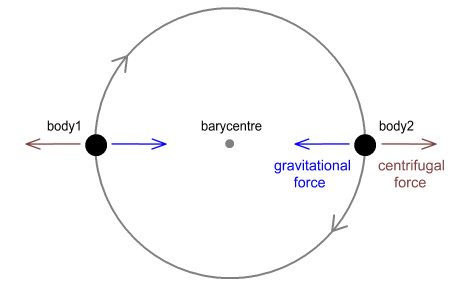They orbit clockwise around a barycentre that’s in the exact middle.  There are two forces at work.  The first is the gravitational force pulling them together and the second is the centrifugal force pulling them apart.

In order to retain their perfect circular orbit it is necessary for these forces to be equal in magnitude, opposite in direction, and perpendicular (at right-angles) to the direction of motion.  If the directions were not like this the bodies would either spiral inwards or outwards.

But why would the force directions not be opposite?

Whenever making predictions about gravity, it is often assumed to be instantaneous, which is to say it is assumed to travel at an infinite speed.  The above diagram also makes this assumption and has the direction of gravitational force pointing directly back at the other body.

But if gravity moved at finite speed this wouldn’t be correct.  Because in the time it took for gravity to reach the other side, the body that emitted the gravity would have moved forward.  Instead, each would see an earlier image of where the other one was.  This diagram shows the idea: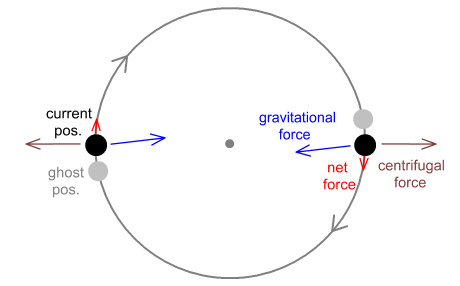Here each body sees a ‘ghost image’ of the other body, where the ‘ghost’ corresponds to an earlier location.  This suggests the gravitational force must be coming from that location rather than the location of where the other body currently is.  So the centrifugal force will still be directly outward but the gravitational force will be at a slight angle.  This means there will now be a net force very mildly in the direction of travel.  This will cause the bodies to spiral slowly outwards: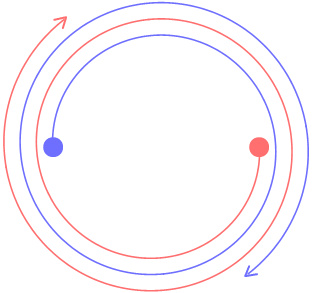In the case where the bodies are of unequal mass, both bodies will be orbiting a shared barycentre and the problem would still occur.  Thus we should likewise expect the Sun and Jupiter to spiral apart, followed by the other planets, leading to the Solar System’s destruction.

#### Tom Van Flandern

This argument was put forward by the late Thomas Van Flandern and can be found here .  Based on this he calculated that, in order to explain the Solar System’s stability, gravity must propagate at at least 2x1010c, i.e. 20 billion times faster than light.   And for all we know it may well propagate at infinite speed allowing it to act instantaneously over any distance.

If gravity really does move that fast it offers exciting opportunities for faster-than-light communication.  On the other hand it also seems unbelievable; especially the idea that it could be instantaneous.

#### Two Archers

But there’s a fault with the above reasoning in that it fails to take into account the motion of the body emitting the gravity.  This can be demonstrated as follows.

Two archers stand on opposing sides of a rotating turntable.  Each has a bow and arrow and is trying to hit the other archer.  Initially they aim directly at where their opponent is standing as shown: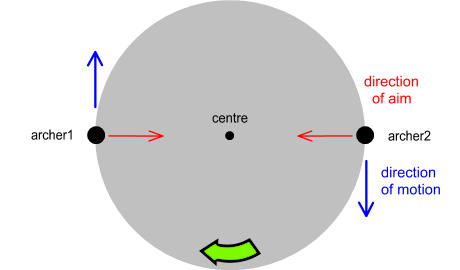The turntable is rotating clockwise.  If the archers release their arrows with this aim they face two problems.  The first is that when the arrow arrives at the other side, the target will have moved.  The second is that the person firing the arrow is moving sideways and this motion will be applied to the arrow.  The end result will be this: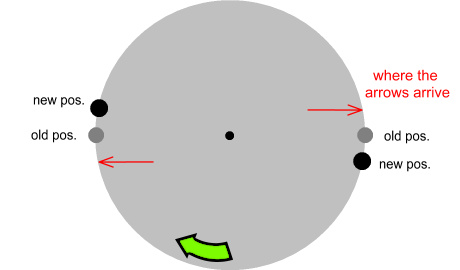Not only do the arrows miss their target, they also miss the location they were aimed at.

In order to compensate for this, each archer must aim to his right as shown: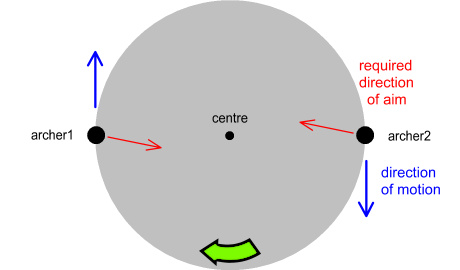When the arrows are released, they will hit their target like this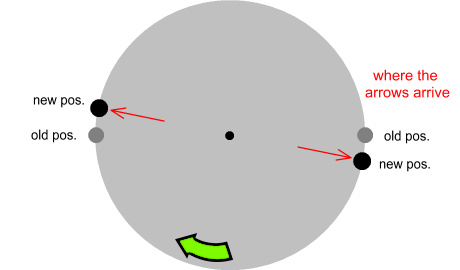Notice how the arrows point back at the archer that fired them.

Now suppose the arrows applied a force to their target and the force direction was along the line that the arrows were pointing.  The archers would feel a force aiming directly away from where they now saw their opponent.  And this would be despite both of them having moved from the spot where the arrows were fired.

Now let’s apply this idea to the original gravity scenario: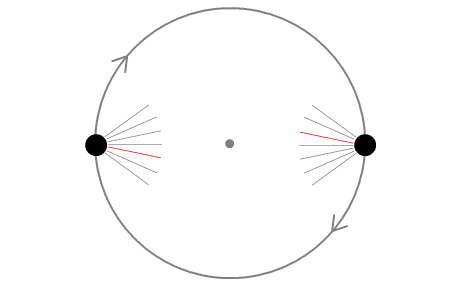Here we see gravity represented as lines radiating from the bodies.  The gravity radiates equally in all directions but only one pair of lines end up hitting the targets.  Those lines are shown in red.

A short while later the gravity hits the opposing bodies.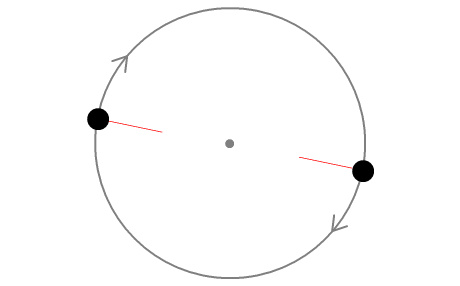Both bodies have moved from their original locations.  But just like the arrows, the red field lines point directly back at the originating bodies’ new locations.

Unlike arrows, gravity exerts an attractive force.  So the net result is a force pointing back at the originating body, and this happens despite there being a delay in the transmission.  The inward force direction is now directly opposite to the centrifugal force and the bodies will not spiral apart after all.

We can apply the same principle to bodies of non-equal mass, such as the Earth-Moon system.  In this case the bodies will move at different speeds with the heavier body moving more slowly and closer to the barycentre.  The below diagram shows the equivalent situations with archers: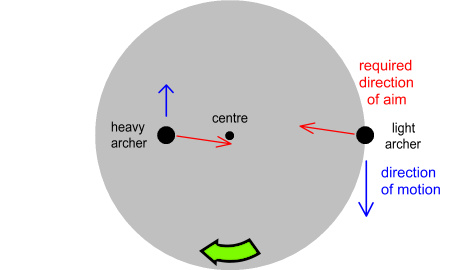Here the ‘heavy’ archer stands closer to the centre of the turntable which represents the barycentre of their ‘orbit’.  But even though their speeds are different (relative to the barycentre), each will use the same angle of aim to hit their target.

Applying this logic to a gravitational situation gives the same result.  Namely that the gravitational force will point back to the originating body, as though gravity had crossed the space between them at infinite speed, even if it hadn’t.

Another reason put forth for why gravity might apply a force in the direction of a body’s motion is Bradley aberration.  In 1727 astronomer James Bradley observed that the location of stars appeared to shift in the direction that Earth moved around the Sun.  This diagram shows the idea: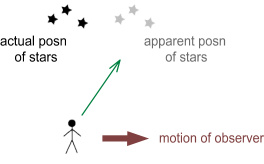It was also noted that the angle the stars were at corresponds to v/c where v is the speed of Earth around the Sun.

If light changes its incoming direction to match tangential velocity, perhaps gravity does likewise?  And perhaps as we orbit the Sun we should also experience gravity at an angle of v/c?

Not necessarily.  In  order to answer this we need to look at what is causing the aberration.  A likely cause of Bradley aberration is that light is hitting the molecules in the atmosphere more on one side than on the other.  This diagram shows the idea: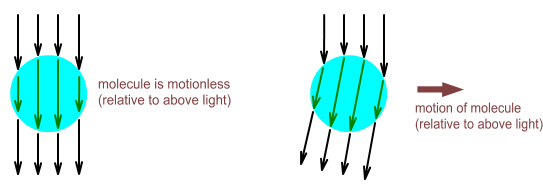The left image shows a molecule as motionless, relative to an above light source.  The light hits the top surface of the molecule equally and gets transmitted directly downward.  In the right image the molecule is moving to the right.  The light hits the right side of the molecule more than the left.  As a result the light is then transmitted at an angle.

Applying this to the atmosphere as a whole, this is most likely what is occurring: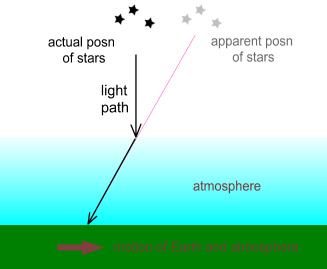Light strikes the atmosphere directly from above but then gets transmitted through the atmosphere at an angle.  This makes the stars appear to be in a different location.  Actually this would occur even if there were no atmosphere because the light would strike a telescope lens or eyeball with a tangential velocity and be transmitted through it at an angle.

So Bradley aberration is likely an optical phenomenon similar to refraction.  We know that light has a number of behaviours when interacting with material.  It can bounce off mirrors, bend through mediums, and diffract through openings.  But we never observe gravity doing these things.  So there is no reason to believe Bradley aberration should necessarily apply to gravity.

#### Further observations on the nature of gravity

What the above tells us is that a slow gravitational speed doesn’t necessarily mean that an orbit will be unstable.  Gravity might move at an infinite speed but that is highly implausible since that would mean its effects would be instantaneous over unlimited distances.  It is also unlikely that it moves at the 2x1010 times faster than light suggested by Van Flandern.

If the above reasoning is correct and gravity does move at a finite speed and without pointing at a ‘ghost image’ of an attracting body, this tells us something about the nature of gravity:

1. That it is radiated at a fixed speed relative to its source rather than to an observer or hypothetical background aether. That is, if gravity moves at speed g (relative to its source) and the source were moving at speed v (relative to an observer), the speed of the gravitational field relative to the observer will be g+v.
2. That the orientation of the field – i.e. the angle it was emitted at – is important and is preserved within the field.
3. That the direction of force will be along the direction that the field was emitted at rather than the direction it impacts a target body.

This diagram shows how gravity acts along the orientation it was emitted at rather than the direction the field is moving at: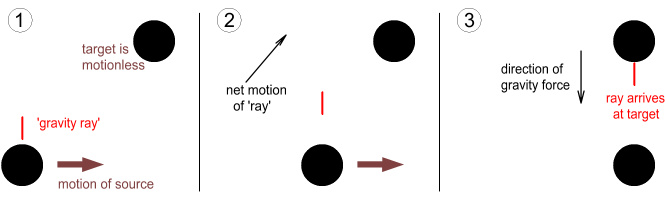Here we see a target body standing motionless and a source moving to the right.  In the first frame the source emits a ‘gravity ray’.  The ray moves directly away from the source whilst also pointing directly away.  In the second frame the ray is halfway to the target and is moving at a diagonal relative to the target.  In the third frame the ray arrives at the target.  Despite it hitting the target at an angle, it applies a force directly downward.

#### Conclusions

The stability of the Solar System cannot be used as proof for gravity being infinite in speed or moving at a large multiple of light-speed.

Bradley aberration is probably an optical effect having to do with light hitting transparent mediums at an angle and is not applicable to gravity.

 Previous Contents Next chapter